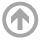Publications

Recent Papers

• 047 Preliminary musings on quantum gravity

This posting is a preliminary draft. Final posting expected by 15 Sept 2010.

• 046 Gravitational waves from the instanton representation of Plebanski gravity.
In this paper we evaluate the instanton representation of Plebanski gravity in the linearized limit. We demonstrate the reproduction of gravitational waves in this limit, obtaining both massless spin-2 polarization modes.

• 045 Gravitational instantons from the instanton representation of Plebanski gravity.
In this paper we formalize the association from the instanton representation to the existence of gravitational instantons for spacetimes of Petrov Types I, D and O where the anti self-dual Weyl curvature (CDJ matrix) is nondegenerate.

• 044 Instanton representation of Plebanski gravity XX: Gravitational coherent states
This paper shows that there exists a natural Hilbert space of harmonic-oscillator coherent states which can be used to describe gravity. Specifically, we provide a prescription for constructing these states corresponding to the kinematic level of the instanton representation of Plebanski gravity,which is obtained by reduced phase space projection from the Ashtekar variables.

• 041 Instanton representation of Plebanski gravity: A brief summary of the classical theory
This paper is a self-contained summary of the instanton representation at the classical level. In this paper we show that starting from the Plebanski theory of gravity, one can obtain two theories of gravity. The first theory is the Ashtekar theory and the second is dual to Ashtekar's theory, where the anti self-dual Weyl curvature is the fundamental momentum space variable. We have called this dual theory the instanton representation. We show how the instanton representation leads to the Einstein equations in the same sense as does the original Plebanski theory, modulo the initial value constraints of GR. The canonical analysis and quantization of the theory is covered in separate papers.

• 042 Instanton representation of Plebanski gravity II: Introduction and duality to the Ashtekar formalism
The Plebanski formulation of gravity is a second-class constrained system which implies the Einstein equations when the equations of motion are satisfied. The elimination of the CDJ matrix from the starting action turns it into a first class constrained system, which includes the Ashtekar formulation of GR as a subset. We have found an action dual to the Ashtekar action called the ‘instanton representation’, which follows upon elimination of the self-dual two forms in favor of the CDJ matrix. We show that the instanton representation implies the Einstein equations, exposes the physical degrees of freedom of GR, and provides a systematic prescription for constructing a solution for nondegenerate metrics. Additionally, we provide a synopsis of various actions which can follow from the starting Plebanski theory.

• 043 Instanton representation of Plebanski gravity III: Classical constraints algebra
We compute the classical algebra of constraints for the instanton representation of Plebanski gravity. The constraints are first class in this representation, with a slightly different structure than in the Ashtekar variables and the metric representation. One main result is that the Hamiltonian constraint forms its own subalgebra in the instanton representation, which has implications for its implementation at the quantum level of the theory.

• 044 Canonical quantization of Plebanski gravity (May 4, 2010)
In this paper we show that the Plebanski theory of gravity implies a theory dual to the Ashtekar variables where the anti self-dual Weyl curvature is the fundamental momentum space variable. The dual theory implies the Einstein equations modulo the initial value constraints, and appears to be consistent in the Dirac sense. Using the dual theory we have obtained a reduced phase space for gravity through  implementation of the initial value constraints. Additionally we have computed the classical dynamics and have performed a quantization on this space, constructing a Hilbert space of states for vanishing cosmological constant. Finally, we have clarified the canonical structure of the dual theory in relation to the Ashtekar theory.